Practice the AP 7th Class Maths Bits with Answers 11th Lesson Area of Plane Figures on a regular basis so that you can attempt exams with utmost confidence.

## AP Board 7th Class Maths Bits 11th Lesson Area of Plane Figures

Multiple Choice Questions :

Question 1.
Area of a rectangle ___________
(A) l × b
(B) l × b × h
(C) 2(l + b)
(D) l + b
(A) l × b

Question 2.
The perimeter of a square =
(A) 6 s
(B) 4 s
(C) s × s
(D) 4 . s × s
(B) 4 s

Question 3.
Perimeter of the triangle whose sides are a, b, c is ___________
(A) $$\frac{1}{2}$$ × b × c
(B) $$\frac{1}{2}$$ × b ×h
(C) a + b + c
(D) a × b × c
(C) a + b + cQuestion 4.
Perimeter of a circle = ___________
(A) 2πr
(B) πd
(C) πr2
(D) A & B
(D) A & B

Question 5.
Area of a right-angled isosceles triangle ___________
(A) Area of rectangle
(B) Area of square
(C) Area of right triangle
(D) None
(B) Area of square

Question 6.
The value of π = ___________
(A) 3.1417
(B) 3.2416
(C) 3.1416
(D) 3.2417
(C) 3.1416

Question 7.
Area of a circular path = ___________
(A) π(R + r) (R – r)
(B) π(R + w) (R – w)
(C) π(2R + 2r)
(D) None
(A) π(R + r) (R – r)

Question 8.
Area of a right angled triangle =
(A) $$\frac{1}{2}$$ × b × h
(B) $$\frac{1}{2}$$ × l × b
(C) $$\frac{1}{2}$$ × a × b
(D) $$\frac{1}{2}$$ × a × r
(C) $$\frac{1}{2}$$ × a × b

Question 9.
Base and height of a triangle are 10 cm and 5 cm, its area is ___________
(A) 25 cm2
(B) 50 cm2
(C) 100 cm2
(D) 30 cm2
(A) 25 cm2

Question 10.
If the side of a square is 7 cm, then its perimeter is cm.
(A) 14
(B) 21
(C) 28
(D) 35
(C) 28

Question 11.
If the area of a rectangle is 180 sq.cm, its length is 20 cm, then its breadth is ___________ cm.
(A) 8
(B) 7
(C) 10
(D)9
(D)9

Question 12.
If the circumference of a circle is 55 cm, then its diameter is ___________ cm.
(A) 17.5
(B) 18
(C) 17
(D) 16.5
(A) 17.5

Question 13.
The area of the shaded path is ___________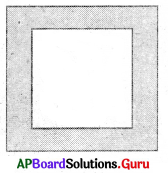(A) Area of inner square – area of outer square
(B) Area of outer square – area of inner square
(C) Area of outer square + area of inner square
(D) None
(B) Area of outer square – area of inner squareQuestion 14.
Length and breadth of a rectangle is 18 cm and 8 cm. If the area of the rectangle equals to the area of a square, then the side of the square is ___________
(A) 8 cm
(B) 12 cm
(C) 16 cm
(D) 18 cm
(B) 12 cm

Question 15.
The length of a rectangle is twice of its breadth, the perimeter is 24 cm, then the length and breadth are ___________
(A) 8 cm, 4 cm
(B) 4 cm, 8 cm
(C) 4 cm, 4 cm
(D) None
(A) 8 cm, 4 cm

Question 16.
Area of the shaded path in the given figure is ___________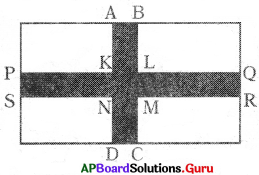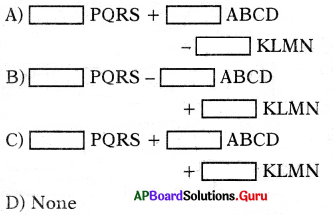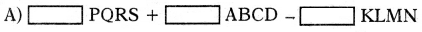Question 17.
The perimeter of the floor of a room is x and its length is y, then its area is ___________
(A) 2xy
(B) xy
(C) 2x + y
(D) y($$\frac{x}{2}$$ – y)
(D) y($$\frac{x}{2}$$ – y)

Question 18.
The circumference of a circle is C. Its area is ___________
(A) $$\frac{4 \pi}{\mathrm{C}}$$
(B) $$\frac{C^{2}}{4 \pi}$$
(C) 2πC2
(D) 4πC2
(B) $$\frac{C^{2}}{4 \pi}$$

Question 19.
The radii of outer and inner circle are 4 cm and 3 cm. Area of the ring is ___________ sq.cm.
(A) 21
(B) 44
(C) 12
(D) 22
(D) 22

Question 20.
The perimeter of a square is 16a. Area of the square is ___________
(A) 4a2
(B) 8a2
(C) 256 a2
(D) 16 a2
(D) 16 a2

Fill in the blanks:

Question 1.
Perimeter of a rectangle is ______
2(l + b)

Question 2.
Area of a square is ______
s × s

Question 3.
Area of a triangle is ______
$$\frac{1}{2}$$ × b × h

Question 4.
Circumference of a circle is ______
2πr (or) πd

Question 5.
Area of a triangle = $$\frac{1}{2}$$ × ______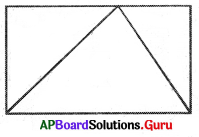Area of the rectangle

Question 6.
The Indian mathematician who calculated V value is ______
Aryabhatta

Question 7.
Area of a circle is ______
πr2 (or) $$\frac{\pi \mathrm{d}^{2}}{4}$$Question 8.
Area of a square path = ______
Area of outer square

Question 9.
Area of the rectangular path = ______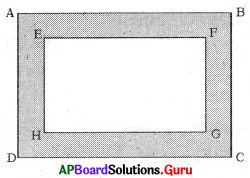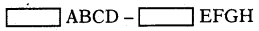Question 10.
The side of a square is 18 cm, then its area is ______
324

Question 11.
The length and breadth of a rectangle are 25 cm and 20 lcm., then its perimeter is ______cm.
90

Question 12.
In the figure BE = ______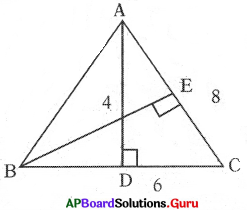3 cm

Question 13.
The radius of a circle is 7 cm, then its circumference is ______
44 cm

Question 14.
If the circumference is 22 cm, then the radius of the circle is ______
3.5 cm

Question 15.
If the perimeter of a square is 36 cm, then its area is ______
81 sq.cm

Question 16.
If l = 15 cm, b = 12 cm, then the perimeter of the rectangle is ______
54

Question 17.
If the height of a triangle is 5 cm and its area is 40 sq.cm., then its base ______
16 cm

Question 18.
The base of a triangle is 40cm ad its area is 220 cm2, then its height is ______ cms.
11

Question 19.
The ratio of circumference of a circle is to its diameter is___________ .
π units

Question 20.
The area of a circle whose radius is7 cm is _____________ sq.cm.
154

Match the following :

Question 1.

 Group A Group B 1. Area of the triangle = ______ (A) 2πr 2. Area of the square = ______ (B) πr2 3. Area of the rectangle = _____ (C) lb 4. Area of the circle = _______ (D) s s 5. Circumference of the circle = _____ (E) $$\frac{1}{2}$$.b.h

 Group A Group B 1. Area of the triangle = ______ (E) $$\frac{1}{2}$$.b.h 2. Area of the square = ______ (D) s s 3. Area of the rectangle = _____ (C) lb 4. Area of the circle = _______ (B) πr2 5. Circumference of the circle = _____ (A) 2πr